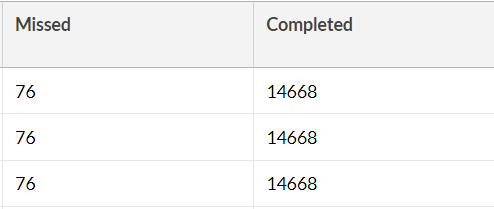# Help with SQL query - Count from a column

Hello everyone,

I'm still beginner in SQL so I would appreciate your help.
I'm trying to write a query that count the number of missed/completed/submitted late assignments for each student in school database.

Please note that I have column called "status":
when
status = 0 --> the assignments is missed.
status = 1 --> the the assignment is completed.
status = 2 --> the assignment is submitted late.

This is my query:

SELECT "student_id" AS 'Student ID',

(SELECT COUNT(status) FROM assignments WHERE status = 0) AS 'Missed',
(SELECT COUNT(status) FROM assignments WHERE status = 1) AS 'Completed',
(SELECT COUNT(status) FROM assignments WHERE status = 2) AS 'Submitted Late',
FROM "assignments"

But the problem with my query is: the result number is not for each specific student.
I mean by that for example, in Missed column I got a number for the total missed assignments for all student in the table, not a specific number for each student.I'm using this query in zoho analytics to create a report.
I tried so many things but I don't know what is my mistake.
If someone can help me I would really appreciate it

Thank you.

https://www.w3schools.com/sql/sql_groupby.asp

https://www.w3schools.com/sql/sql_ref_case.asp

``````SELECT student_id
,SUM(CASE WHEN [status] = 0 THEN 1 ELSE 0 END) AS Missed
,SUM(CASE WHEN [status] = 1 THEN 1 ELSE 0 END) AS Completed
,SUM(CASE WHEN [status] = 2 THEN 1 ELSE 0 END) AS SubmittedLate
FROM Assignments
GROUP BY student_id``````
1 Like

That solved my problem
Thank you very much !!